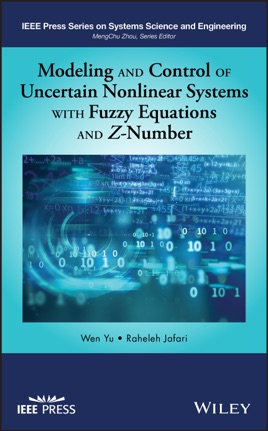• \$79.99

## Publisher Description

An original, systematic-solution approach to uncertain nonlinear systems control and modeling using fuzzy equations and fuzzy differential equations

There are various numerical and analytical approaches to the modeling and control of uncertain nonlinear systems. Fuzzy logic theory is an increasingly popular method used to solve inconvenience problems in nonlinear modeling. Modeling and Control of Uncertain Nonlinear Systems with Fuzzy Equations and Z-Number presents a structured approach to the control and modeling of uncertain nonlinear systems in industry using fuzzy equations and fuzzy differential equations.

The first major work to explore methods based on neural networks and Bernstein neural networks, this innovative volume provides a framework for control and modeling of uncertain nonlinear systems with applications to industry. Readers learn how to use fuzzy techniques to solve scientific and engineering problems and understand intelligent control design and applications. The text assembles the results of four years of research on control of uncertain nonlinear systems with dual fuzzy equations, fuzzy modeling for uncertain nonlinear systems with fuzzy equations, the numerical solution of fuzzy equations with Z-numbers, and the numerical solution of fuzzy differential equations with Z-numbers. Using clear and accessible language to explain concepts and principles applicable to real-world scenarios, this book:
Presents the modeling and control of uncertain nonlinear systems with fuzzy equations and fuzzy differential equations Includes an overview of uncertain nonlinear systems for non-specialists Teaches readers to use simulation, modeling and verification skills valuable for scientific research and engineering systems development Reinforces comprehension with illustrations, tables, examples, and simulations
Modeling and Control of Uncertain Nonlinear Systems with Fuzzy Equations and Z-Number is suitable as a textbook for advanced students, academic and industrial researchers, and practitioners in fields of systems engineering, learning control systems, neural networks, computational intelligence, and fuzzy logic control.